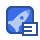# 使用案例学习LOF算法

4星（超过85%的资源）371收藏

LOF (Local Outlier Factor) Example Consider the following 4 data points a(0,0),b(0,1),c(1,1),d(3,0) Calculate the lof for each point and show the top 1 outlier, set k=2 and use Manhattan Distance Step 1: calculate all the distances between each two data points There are 4 data points a(O,O),b(0,1),c(1,1),d(3,0) (Manhattan Distance here dist(a, b=1 dist(a, c)=2 dist(a, d ) =3 dist(b, c)=1 dist(b,d)=3+1=4 dist(c,d)=2+1=3 Step 2 calculate all the dist(a, c)=2 c is the 2nd nearest neighbor) = dist(b, a)=1(a/c is the 2nd nearest neighbor) dist(c, a=2 (a is the 2nd nearest neighbor) dist(d, a)=3(a/c is the 2nd nearest neighbor) Step 3: calculate all the oinD,(o,o)≤ ={a,c} Step 4 calculate all the N Irak(o) o′∈Nk(o) eachdisth(o r'eachdisth(o+o)=max distr(o), dist(o, o)1 reachdist be-a)+reachdist,( cea Step 4 calculate all the r'eachdistK(o+o)=max disth(o), dist(o,O) reachdist2(b(a)=max(dist2(b), dist(b, a)) reachdist (c< a)=max dist(c), dist(c, a) I N2(a)ll reachdist, (be-a)+reachdist2 (ce-al Step 4: calculate all the N2(b) reachdist2(aeb)+reachdist2(ct-b) N2c reachdist, (be-C)+reachdist2 aec N2(b) reachdist2lae-d)+reachdist2 ced Step 5: calculate all the rake LOFkO o∈Nk(O)rdk(O) ∑llk(o)·∑ reachdistk(=) ∈Nk( ∈Nk(O) reachdist2(b←a) reachdist2(c←a reachdist(a←b) reachdist2(Cc←b

...展开详情

2018-04-05nujnujouhz 其实就是这个地址：http://www.cse.ust.hk/~leichen/courses/comp5331/lectures/LOF_Example.pdf
2017-05-10

2017-03-16

2016-12-08

2016-08-16

2016-04-24nalanding 很不错的东西，写的很详细，就是参照这个写出代码来了，建议下载
2016-03-26

• 4
资源
• 0
粉丝
•等级使用案例学习LOF算法 50积分/C币 立即下载
1/1250积分/C币 立即下载